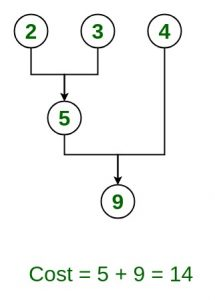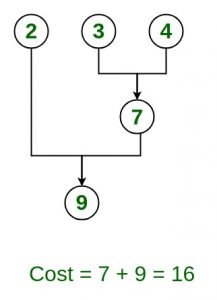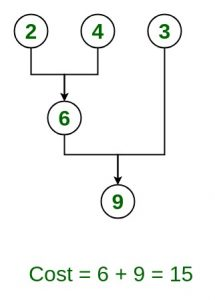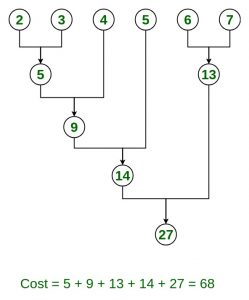Skip to content
Related Articles
Optimal File Merge Patterns
• Difficulty Level : Medium
• Last Updated : 27 Aug, 2020

Given n number of sorted files, the task is to find the minimum computations done to reach Optimal Merge Pattern.

When two or more sorted files are to be merged all together to form a single file, the minimum computations done to reach this file are known as Optimal Merge Pattern.

If more than 2 files need to be merged then it can be done in pairs. For example, if need to merge 4 files A, B, C, D. First Merge A with B to get X1, merge X1 with C to get X2, merge X2 with D to get X3 as the output file.

If we have two files of sizes m and n, the total computation time will be m+n. Here, we use greedy strategy by merging two smallest size files among all the files present.

Examples:
Given 3 files with size 2, 3, 4 units.Find optimal way to combine these files

Input: n = 3, size = {2, 3, 4}
Output: 14
Explanation: There are different ways to combine these files:
Method 1: Optimal methodMethod 2:Method 3:Input: n = 6, size = {2, 3, 4, 5, 6, 7}
Output: 68
Explanation: Optimal way to combine these filesApproach:

Node represents a file with a given size also given nodes are greater than 2

1. Add all the nodes in a priority queue (Min Heap).{node.weight = file size}
2. Initialize count = 0 // variable to store file computations.
3. Repeat while (size of priority Queue is greater than 1)
1. create a new node
2. new node = pq.poll().weight+pq.poll().weight;//pq denotes priority queue, remove 1st smallest and 2nd smallest element and add their weights to get a new node
3. count += node.wight
4. add this new node to priority queue;
4. count is the final answer

Below is the implementation of the above approach:

## C++

 `// C++ program to implement``// Optimal File Merge Pattern``#include``using` `namespace` `std;`` ` `// Function to find minimum computation ``int` `minComputation(``int` `size, ``int` `files[])``{``     ` `    ``// Create a min heap``    ``priority_queue<``int``, vector<``int``>, ``           ``greater<``int``>> pq;`` ` `    ``for``(``int` `i = 0; i < size; i++)``    ``{``         ` `        ``// Add sizes to priorityQueue``        ``pq.push(files[i]);``    ``}``     ` `    ``// Variable to count total Computation``    ``int` `count = 0;`` ` `    ``while``(pq.size() > 1)``    ``{``         ` `        ``// pop two smallest size element``        ``// from the min heap``        ``int` `first_smallest = pq.top();``        ``pq.pop();``        ``int` `second_smallest = pq.top();``        ``pq.pop();``         ` `        ``int` `temp = first_smallest + second_smallest;`` ` `        ``// Add the current computations``        ``// with the previous one's``        ``count += temp;`` ` `        ``// Add new combined file size``        ``// to priority queue or min heap``        ``pq.push(temp);``    ``}``    ``return` `count;``}`` ` `// Driver code``int` `main()``{``     ` `    ``// No of files``    ``int` `n = 6;``     ` `    ``// 6 files with their sizes``    ``int` `files[] = { 2, 3, 4, 5, 6, 7 };``     ` `    ``// Total no of computations``    ``// do be done final answer``    ``cout << ``"Minimum Computations = "``         ``<< minComputation(n, files);`` ` `    ``return` `0;``}`` ` `// This code is contributed by jaigoyal1328`

## Java

 `// Java program to implement``// Optimal File Merge Pattern`` ` `import` `java.util.Scanner;``import` `java.util.PriorityQueue;`` ` `public` `class` `OptimalMergePatterns {`` ` `    ``// Function to find minimum computation``    ``static` `int` `minComputation(``int` `size, ``int` `files[])``    ``{`` ` `        ``// create a min heap``        ``PriorityQueue pq``            ``= ``new` `PriorityQueue<>();`` ` `        ``for` `(``int` `i = ``0``; i < size; i++) {`` ` `            ``// add sizes to priorityQueue``            ``pq.add(files[i]);``        ``}`` ` `        ``// variable to count total computations``        ``int` `count = ``0``;`` ` `        ``while` `(pq.size() > ``1``) {`` ` `            ``// pop two smallest size element``            ``// from the min heap``            ``int` `temp = pq.poll() + pq.poll();`` ` `            ``// add the current computations``            ``// with the previous one's``            ``count += temp;`` ` `            ``// add new combined file size``            ``// to priority queue or min heap``            ``pq.add(temp);``        ``}`` ` `        ``return` `count;``    ``}`` ` `    ``public` `static` `void` `main(String[] args)``    ``{`` ` `        ``// no of files``        ``int` `size = ``6``;`` ` `        ``// 6 files with their sizes``        ``int` `files[] = ``new` `int``[] { ``2``, ``3``, ``4``, ``5``, ``6``, ``7` `};`` ` `        ``// total no of computations``        ``// do be done final answer``        ``System.out.println(``"Minimum Computations = "``                           ``+ minComputation(size, files));``    ``}``}`
Output:
```Minimum Computations = 68
```

Attention reader! Don’t stop learning now. Get hold of all the important DSA concepts with the DSA Self Paced Course at a student-friendly price and become industry ready.  To complete your preparation from learning a language to DS Algo and many more,  please refer Complete Interview Preparation Course.

In case you wish to attend live classes with industry experts, please refer Geeks Classes Live

My Personal Notes arrow_drop_up